## Understanding Table Calcs using Index()

I’ve been a Tableau user for two years now. Since joining The Information Lab a few weeks ago I decided it was about time I stopped being scared of table calculations and started understanding them more.

One of the biggest hurdles of table calcs is understanding just what they are doing with your data and what the difference between using a Table (across), a Pane (across) or any of the other ‘compute using’ options is.

### How Tableau computes table calculations

When we select a table calc, Tableau first queries our data source (whether that be a database connection, extract, or spreadsheet). The query then returns the data for the table calc in a temporary table stored in cache. Tableau can then reference this temporary table when it needs to. Watch Robert Morton’s presentation on Tableau’s Query Cache for a thorough explanation.

Okay, so now we have a brief understanding of what Tableau is doing to create a table calc, let’s go ahead and connect to Superstore Sales in Tableau Desktop and start creating some table calcs.

I’ve placed Year and Month of Order Date on the columns shelf, and Sum of Sales on rows. Now add your table calc by right-clicking Sum(Sales) and select Quick Table Calculation – Running Total.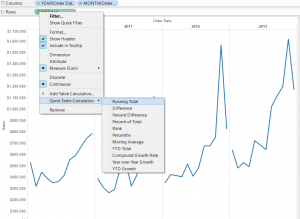So, pretty straight forward here. Tableau is totalling Sum(Sales) for every month across our chart. Let’s understand what Tableau is doing a bit better by using the Index() (or rank) calculation.

Create a new calculated field with the following: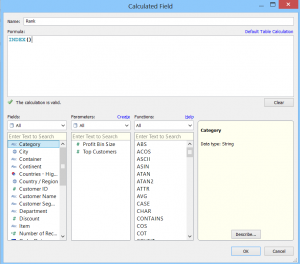Now place your new calculated field on to Label. Notice that Rank is also a table calc! If you’ve never used Index() before, this is a great little function that can be used to create a rank – although if you’re already using Tableau 8.1 you now have some ranking table calculations to choose from. If you would like to learn more about the Index() function have a look at the series of videos that Tom Brown made here.

Okay, so now we have some numbers on our line chart: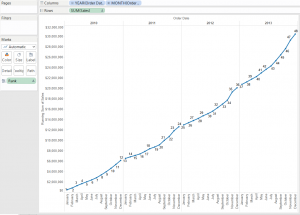The Rank calculated field is showing us exactly how our table calculation is working. Tableau is going across the table starting in January 2010 and ending in December 2013, totalling Sales month by month.

### Okay, let’s mix things up a bit

Now change the ‘compute using’ for our table calculation to Pane (across) and make sure you change your Rank calculation compute to do the same.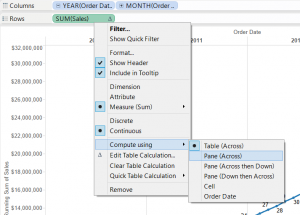Now the Rank calculated field becomes really useful to see how Tableau has changed what it is addressing in our table calc. Using Pane (across) makes Tableau address every Month and partition (or restart) the table calculation every Year from left to right across the chart. Now we get a running total of Sales which restarts every year.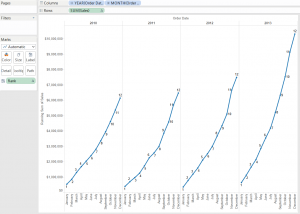Now try using the Rank calculated field on some different Table Calcs to really understand what each ‘compute using’ does.

### Year over Year Growth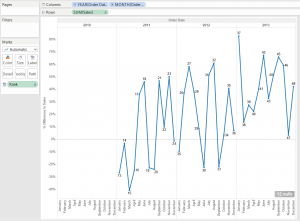Here Tableau is comparing growth to the previous year. As 2010 is our first year, it will be null as we have nothing previous to compare to. The table calc then draws our first mark in Jan 2011. This is showing us the growth (or minus growth in this case) from Jan 2010. It then goes to the next mark in Feb 2011 and marks the growth compared to Feb 2010, and so on.

### Moving Average, compute using Pane (Across then Down)

This time I’ve create a cross tab with a moving average table calc on Sales, then set the ‘compute by’ to Pane (across then down).  Here the Rank label shows us that Tableau is calculating the moving average by working across the pane (calculating all the moving averages across the years for bookcases) and then moving down to the next category.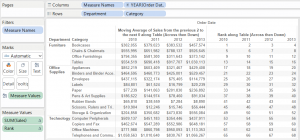Hopefully by now Tableau table calcs, the compute using function and how this changes what Tableau addresses and partitions by is making a bit more sense.

Go ahead and take this a step further and learn how to use the advanced table calculation options; check out Tom’s blog post here.

London, UK